# Enhancing images using Python: An Image Processing Introduction

In my previous article, I discussed how we could a little bit more understand a digital image using Python. Now I will discuss how we will be able to enhance digital images still using Python via White Balancing and Histogram Manipulation.

# White Balancing

White balancing is a method where we correct a digital image by turning white or neutral colored regions appear white in a digital image. I will discuss three algorithms of white balancing: white patch, gray world, and ground-truth. For the sake of simplicity, let’s reuse the image from my previous article: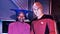Guinan & Captain Jean-Luc Picard aboard USS Enterprise-D

## White patch algorithm

This algorithm normalizes each color channel depending on the maximum allowed value within that specific channel in order to enhance the image. So, let’s look at the histogram of each of our pixel values using the following code:

`import numpy as npimport skimage.io as skiofrom skimage import img_as_ubyte, img_as_floatguinanpicard = skio.imread(‘guinanpicard.jpg’)for channel, color in enumerate(‘rgb’):    channel_values = guinanpicard[:,:,channel]    plt.step(np.arange(256),     np.bincount(channel_values.flatten(), minlength=256)*1.0/channel_values.size, c=color)    plt.xlim(0, 255)    plt.axvline(np.percentile(channel_values, 95), ls=’ — ‘, c=color)    plt.xlabel(‘channel value’)    plt.ylabel(‘fraction of pixels’);`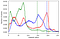Histogram of pixel values in RGB color channel

We could see from above that the maximum value of each of the color channels are at 255, however this also means that applying a direct white patch to our image would not change the results. So rather than using the maximum value, we will use the 95th percentile of their values for the white patch:

`guinanpicard_wp = img_as_ubyte((guinanpicard * 1.0 / np.percentile(guinanpicard, 95, axis=(0, 1))).clip(0, 1))skio.imshow(guinanpicard_wp)`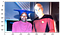Resulting image from the white-patch algorithm

The resulting white-patched image seems to be a bit “brighter” compared the original one, but we could see here that the face of Picard is too bright. Try playing with the percentile values to get better results.

## Gray world algorithm

The next algorithm on our list is the gray-world algorithm. It assumes that pixels are gray on average. This means that for every green pixel, there is a red or blue pixel somewhere within the image which means that the mean value for each color channel should be the same. Therefore, we will adjust each color channel so that they have the same mean values:

`guinanpicard_gw = ((guinanpicard* (guinanpicard.mean() / guinanpicard.mean(axis=(0, 1)))).clip(0, 255).astype(int))skio.imshow(guinanpicard_gw);`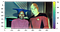Resulting image from the gray world algorithm

## Ground truth algorithm

The last white balancing algorithm that we will implement is the ground-truth algorithm. The difference between the ground truth to other algorithms is that we do not assume that the brightest spots should be white or on average an image is gray, but rather we will choose a patch within the image where we know it should be “true” white and use that to rescale our color channels:

`from matplotlib.patches import Rectangle## Showing the region where the patch is basedfig, ax = plt.subplots()ax.imshow(guinanpicard)ax.add_patch(Rectangle((618, 250), 25, 25, edgecolor='r', facecolor='none'));## Extracting the patchgp_patch = guinanpicard[250:275, 618:633]skio.imshow(gp_patch);`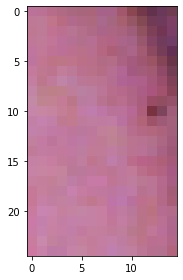Original image (left) showing where the patch (right) is extracted

I chose a well-lit area of the wall behind where Picard is standing as the “true” white patch area of the original image. We will now use this to normalize our image either via getting the maximum (similar to white patch) or mean (similar to gray world) values of the color channels:

`## Getting maximum valuegp_gt_max = (guinanpicard *1.0 / gp_patch.max(axis=(0, 1))).clip(0, 1)skio.imshow(gp_gt_max)## Getting the mean valuegp_gt_mean = ((guinanpicard * (gp_patch.mean() / guinanpicard.mean(axis=(0, 1)))).clip(0, 255).astype(int))skio.imshow(gp_gt_mean);`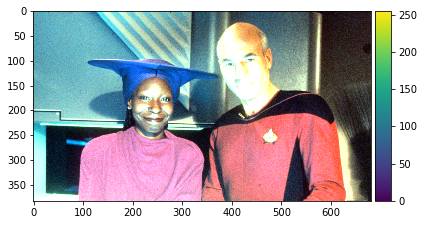Resulting image using max values (left) and mean values (right)

It is quite obvious that the image on the left is brighter and somewhat better compared the one the right. We could also see other colors coming out from the image compared to the original one which has a red hue.

# Histogram Manipulation

Histogram manipulation is an image enhancement technique used to improve images that are (on this case) under exposed. Normally, this could be done easily by image editing software available but let’s try implementing this manually. For this one, I will use an image I got on Google: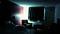A not well lit room

As you can see the room is not, well, lit. So we will use histogram manipulation in order to see room much better, but first we need to know the intensity values of the pixels within the image:

`import matplotlib.pyplot as pltfrom skimage.color import rgb2grayfrom skimage.exposure import histogram, cumulative_distributiondark_room_intensity = img_as_ubyte(rgb2gray(dark_room))freq, bins = histogram(dark_room_intensity)plt.step(bins, freq*1.0/freq.sum())plt.xlabel(‘intensity value’)plt.ylabel(‘fraction of pixels’);`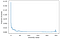Distribution of intensity values

It is obvious that most of the pixels in the image have very low intensity values. We need to make the distribution uniform, but first we need to get both the actual and target CDF of the pixels. After getting the two CDFs, we will compute the percentile of each intensity from the actual CDF and use the corresponding value in having the same percentile within the target CDF:

`## Getting the actual vs target CDFfreq, bins = cumulative_distribution(dark_room_intensity)target_bins = np.arange(255)target_freq = np.linspace(0, 1, len(target_bins))plt.step(bins, freq, c=’b’, label=’actual cdf’)plt.plot(target_bins, target_freq, c=’r’, label=’target cdf’)plt.plot([50, 50, target_bins[-11], target_bins[-11]], [0, freq, freq, 0],  ‘k — ‘,  label=’example lookup’)plt.legend()plt.xlim(0, 255)plt.ylim(0, 1)plt.xlabel(‘intensity values’)plt.ylabel(‘cumulative fraction of pixels’);`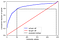Chart comparing the actual and target CDF

After that we can now proceed in enhancing the image using the following code:

`new_vals = np.interp(freq, target_freq, target_bins)dark_room_eq = img_as_ubyte(new_vals[dark_room_intensity].astype(int))imshow(dark_room_eq);`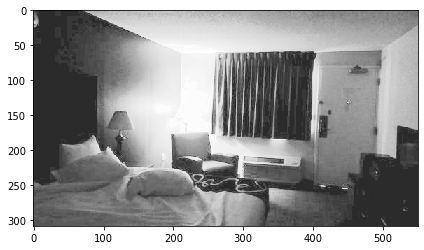Comparison between the original image (left) and the enhanced image (right)

As you could see, we were able to “brighten up” the not so-well lit room. We could identify also now identify what the room really looks like if it’s well lit. We now also know where the bed is located, how many pillows are in the bed, and even how the door looks like from the inside.

And there you have it! These are the two ways on enhancing our digital image, however there are a lot of ways in doing this that we haven’t discussed. For example, the histogram manipulation implementation is mainly focused on histogram equalization, but you could also implement this via contrast stretching.

A developer by day, a programmer by night

## More from Cymon Marcaida

A developer by day, a programmer by night